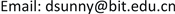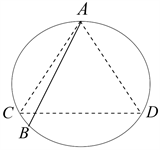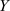﻿ 关于概率空间的思辨教学 Speculative Teaching on Probability Space

Vol. 10  No. 01 ( 2020 ), Article ID: 33935 , 5 pages
10.12677/AE.2020.101012

Speculative Teaching on Probability Space

Yang Zhao

School of Mathematics and Statistics, Beijing Institute of Technology, BeijingReceived: Dec. 24th, 2019; accepted: Jan. 7th, 2020; published: Jan. 14th, 2020ABSTRACT

Probability space is an important concept in probability theory and a platform for all knowledge and problems. This paper explains the origin of probability space through speculative teaching, and uses different ways of building probability space to solve problems, which is conducive to cultivating students’ multi-dimensional thinking ability.

Keywords:Probability Space, Speculative Teaching, Bertrand Paradox1. 引言

2. 概率空间概念教学的追根求源

$E$ 是随机试验， $S$ 是它的样本空间， $\sigma$ 是某些子集的集合，对于 $\sigma$ 的每一个事件 $A$ 赋予一个实数，记为 $P\left(A\right)$，若 $P\left(A\right)$ 满足下列三个条件：1) 非负性：对每一个事件 $A$，有 $P\left(A\right)\ge 0$；2) 完备性： $P\left(S\right)=1$；3) 可列可加性：设 ${A}_{1},{A}_{2},\cdots$ 是两两互不相容的事件，则有 $P\left({\cup }_{i=1}^{\infty }{A}_{i}\right)=\underset{i=1}{\overset{\infty }{\sum }}P\left({A}_{i}\right)$。则称 $P\left(A\right)$ 为事件 $A$ 的概率，( $S$, $\sigma$, P)为一概率空间。这样的讲述有两个问题被回避了：概率作为从集合到数的映射具有很多性质，为什么在定义中只考察这三种性质，特别是可列可加性？为什么概率定义在S的部分子集上？而不是全部子集上？虽然严格的解释需要测度论，但仍然可以给学生一个引导，来分析是如何思考和解决新问题的。在这里可以尝试做了如下解释：集合到数的映射给出了对集合的一种度量，比如区间的长度，平面区域的面积，空间区域的体积，都可以看作是对集合的一种度量，这个度量可以在纵横两方面推广，直线上比区间更复杂的点集是否可有“长度”的概念？n维空间的点集的度量是什么？这就引入测度的概念，用来度量一个集合E的“大小”，记为m(E)，作为长度，面积，体积的一种推广方式，自然要求满足一定的条件才合理，比如m作用在区间上仍然是长度，具有长度已有的性质：有限可加性，可列可加性。但这些限制无法使得一切点集合都有度量，从而对点集的结构有一定要求，在一类特殊的子集上可以定义满足条件的测度。而这个概率就是一种归一化的测度。这样的解释虽然不够严格，却给出了直观的思维方式，理解程度高的同学可以进一步去读读测度论，从而拓展知识的宽度和深度。

3. 概率空间概念教学的应用案例

$P\left(A\right)=\frac{4}{10}$,$P\left(\stackrel{¯}{A}B\right)=\frac{6\ast 4}{10\ast 9}$,$P\left(ABC\right)=\frac{4\ast 3\ast 2}{10\ast 9\ast 8}$.

$P\left(A\right)=\frac{4}{10}$,$P\left(\stackrel{¯}{A}B\right)=\frac{6\ast 4}{10\ast 9}$.

ABC不是这个样本空间的子集，不能直接在此模型下计算。

$P\left(\stackrel{¯}{A}B\right)=P\left(\stackrel{¯}{A}\right)P\left(B/\stackrel{¯}{A}\right)=\frac{6}{10}\ast \frac{4}{9}$$P\left(ABC\right)=P\left(A\right)P\left(B/A\right)P\left(C/AB\right)=\frac{4}{10}\ast \frac{3}{9}\ast \frac{2}{8}$Figure 1. B is the uniform distribution on the circleFigure 2. B is the uniform distribution on the diameter

$X:\Omega \to R,\left(a,b\right)↦a,Y:\Omega \to R,\left(a,b\right)↦b,$

$X,Y$ 相互独立，都服从 $\left(0,2\pi \right]$ 上的均匀分布。记E为满足题目条件的样本点的集合，在两维的情况下P(E)不太好确定，利用下面的连续型全概率公式  转化一维情形。

$X$ 的密度函数为 $f\left(x\right)=\left\{\begin{array}{cc}\frac{1}{2\pi }& x\in \left(0,2\pi \right]\\ 0& 其它\end{array}$，对于 $x\in \left(0,2\pi \right]$，在 $X=x$ 条件下，的条件密度函数为 ${f}_{Y/X}\left(y/x\right)=\left\{\begin{array}{cc}\frac{1}{2\pi }& y\in \left(0,2\pi \right]\\ 0& 其它\end{array}$，则 $P\left(E/X=x\right)=\frac{1}{3}$，根据上面定理可得： $P\left(E\right)={\int }_{0}^{2\pi }\frac{1}{2\pi }\ast \frac{1}{3}dx=\frac{1}{3}$。这里就利用概率空间的概念严格解释了解法1的正确性。

4. 总结

Speculative Teaching on Probability Space[J]. 教育进展, 2020, 10(01): 71-75. https://doi.org/10.12677/AE.2020.101012

1. 1. 蔺云. 珍视概率统计课程中思辨数学的教育价值[J]. 数学教育学报, 2010, 19(3): 18-21.

2. 2. 盛骤, 谢式千, 潘承毅. 概率论与数理统计[M]. 第四版. 北京: 高等教育出版社, 2008.

3. 3. 王寿仁. 概率论概貌[M]. 北京: 科学技术文献出版社, 1991.

4. 4. 苏同安. 都是圆心惹的祸——“贝特朗悖论”新说[J]. 中学数学高中版, 2010(1): 64.

5. 5. 徐明. “几何概型”教学释疑——兼谈“贝特朗概率悖论” [J]. 数学通讯, 2009(6): 15-17.

6. 6. 张敏, 何小亚. 贝特朗悖论之争的终结[J]. 数学教育学报, 2015, 24(3): 51-54.

7. 7. 黄字瀚, 褚人统. 也习贝特朗概率悖论再研几何概率模型[J]. 数学通报, 2014, 53(9): 24-27.

8. 8. 辛玉东, 毕建芝. 连续型全概率公式及其应用[J]. 中国教育教学杂志(高等基育版), 2006, 12(145): 91-92.